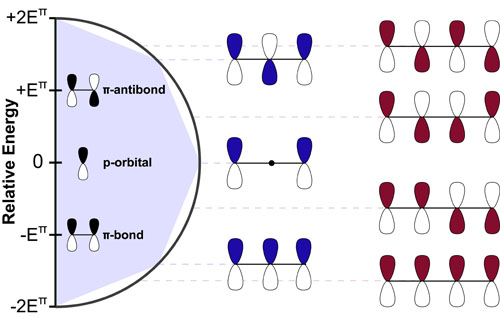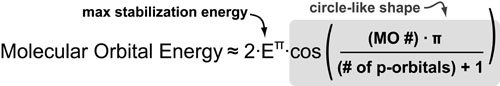# The Energies of Linear Frontier Molecular OrbitalsThe Woodward Hoffman rules are some of the most useful rules in organic chemistry. Unfortunately, because these rules are symmetry-based, they mostly ignore the relative energies of the molecular orbitals they consider. Luckily, Huckel theory (on which the Wood-ward Hoffman rules were based) gives as simple, geometric handle on the energies of these orbitals. Understanding these energies is critical for (1) rationalizing non-pericyclic reactivity trends and (2) answering the question: What exactly is aromaticity?

In general, there is a maximum stabilization/destabilization energy associated with conjugated pi systems which is equal to 2 times the stabilization energy of a single pi-bond (2Eπ). This is the coefficient of the equation derived by Huckel below. In linear systems, this maximum is never reached but is asymptotically approached by the lowest energy molecular orbital.

The distribution of molecular orbitals between the minimum and maximum energies is defined by the cosine term in the equation below which gives this distribution a circular shape. A simple geometric trick to derive these energies is to embed an equilateral shape with 2x+2 sides (where x is the number of p-orbitals) into this circle with a vertex pointed down.### REFERENCES:

1. McBride, J.M. Yale Chem 125: Freshman Organic Chemistry
2. Huckel, E. Zeitschrift fur Physik, 1931, 70 204.
3. Frost, A. A.; Musulin, B. Mnemonic device for molecular-orbital energies. J. Chem. Phys. 1953. 21, 572–573.
4. Brown, A.D.; Brown, M. D. A geometric method for determining the Huckel molecular orbital energy levels of open chain, fully conjugated molecules. J. Chem. Educ. 1984, 61, 770.
5. Fleming, I. Frontier Orbitals and Organic Chemical Reactions, Wiley-Interscience, 2004
6. McQuarrie, D.A.; Simon, J.D. Physical Chemistry: A Molecular Approach. University Science Books, 1997